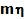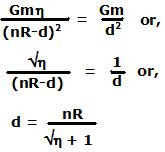## Monday, November 10, 2008

### Irodov Problem 1.231Let the mass of Moon be m, the the mass of Earth is. Let point O be the point where the gravitational pull of the Earth exactly equals that of the Moon. R is the radius of the Earth. Let d be the distance of O from the center of the Moon. At point O the gravitational force field due to Earth and the Moon must be exactly equal and so we have,